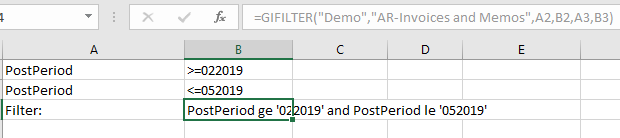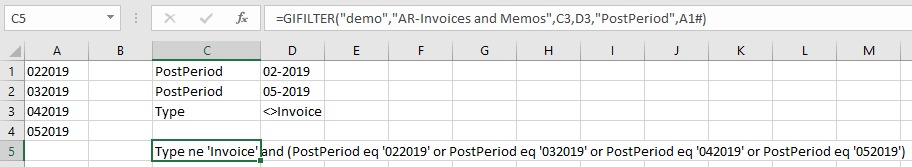Portal Categories Articles in this folder You may like to read

# Filtering Generic Inquiries by financial period range

Created by Damien Zwillinger, Modified on Tue, 12 Sep 2023 at 04:08 AM by Damien Zwillinger

Applies to:

• Velixo NX
• Velixo Classic < 6.0.411
• Acumatica, Cegid, MYOB

## Overview

When working with Generic Inquiries...... there are times when it is necessary to filter on financial period, specifically a range of financial periods.

In the above example, however, if we wanted to show only those PostPeriod between 022019 and 052019:...this would not work correctly.

While this method works for dates, the PostPeriod data is simply an alphanumeric string of text which `ge` and `le` operators compare "lexicographically", i.e. in the sort order of words in a dictionary.

Thus, If we use this technique, we'll get all "words" between "022019" and "052019", which would include "032013", "042016", "052018", etc. Obviously, this is not what we want.

So, in this example, what we need to do is to come up with a way for our filter to state: "We want period 022019, 032019, 042019, and 052019". (and it would be really nice if it would work for ANY number of periods).

We *could* simply type the filter we need (if we know how to correctly format it). Thus, we could type:

PostPeriod eq '022019' or PostPeriod eq '032019' or PostPeriod eq '042019' or PostPeriod eq '052019'

This would work, but we would have to re-type it every time we wanted a different range.

## Step-by-Step

So, the first thing we need is a list of the periods.

By putting our start and end periods in cells D2 and D3, we can then use the FINANCIALPERIODLIST function in cell A1 to give us all the periods in that range.We'll also need to remove the dash "-" from the periods (we can see in the data that the Generic Inquiry leaves it out). This is done by embedding the Velixo function in Excel's SUBSTITUTE function.

Now, we can use the Velixo GIFILTER function. We put whatever other filters we need (in this case we only want non-invoices) along with the name of our period field ("PostPeriod) and a reference to our list of dates in cell A1 (we need to the # at the end of that reference in order to get *all* of the values).

The GIFILTER function automatically formats the filter (including using the correct parentheses) for the values we need:We'll reference that filter in our GI function and, now, we only get the periods we want:While not trivial, it's just a few simple functions to format the filter in a way that the Acumatica Generic Inquiry needs.